# CREST Olympiads - CRO PDF Sample Papers for Class 3

Class 3 sample paper & practice questions for CREST Reasoning Olympiad (CRO) level 1 are given below. Syllabus for level 1 is also mentioned for these exams. You can refer these sample paper & quiz for preparing for the CRO exam.#### Resources:

##### Sample Questions from Olympiad Success:
 Q.1 Q.2 Q.3 Q.4 Q.5 Q.6 Q.7 Q.8 Q.9 Q.10
 Q.1 Four books are kept in a column, one above the other. Maths book is kept above English book. Science book is kept below Hindi book. Which book is kept at the bottom? a) English b) Science c) Hindi d) Cannot be determined
 Q.2 How many N's are there in the following series which are immediately preceded by M and also immediately followed by M?M M N M N M E N M N F P N M N M N M N M a) 3 b) 5 c) 2 d) None of these above
 Q.3 There are four bags labelled as A, B, C and D. Each of them has different number of balls. B has more balls than D. A has more balls than B. C has the least number of balls i.e. two. A has three more balls than C. Which of the following bags has four balls? a) B b) A c) D d) Cannot be determined
 Q.4 Find out the next figure of the following series: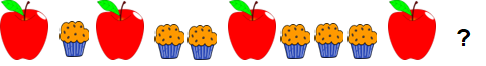a)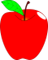b)c)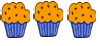d)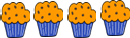Q.5 In a certain code, PIZZA is coded as #@##@, BURGER is coded as #@##@#, then TOFFEE will be coded as: a) @#@##@ b) #@#@## c) ##@#@@ d) #@##@@
 Q.6 Which of the following figure has the same number of corners as the figure below?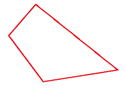a)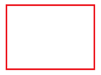b)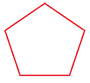c)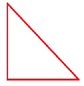d)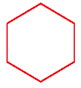Q.7 Find out the next figure of the following series: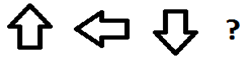a)b)c)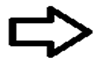d)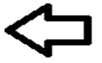Q.8 Naman wants to go to school from his house. First of all, he goes to the crossing, from here he turns to right and reaches the bus stand. Bus stand is opposite to the library. In which direction is the school located? a) North b) East c) North-West d) Cannot be determined
 Q.9 If letter N represents North, E represent East, W represents West and S represents South and dots represents the number of steps, then find the code for the path given below: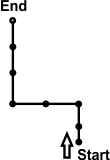a) N2E2N3 b) S3E2S2 c) N2W2N3 d) S3W2S3
 Q.10 Find the next letter of the following series: A, C, E, G, ? a) K b) J c) H d) ISample PDF of CREST Olympiads - CREST Reasoning Olympiad (CRO) PDF Sample Papers for Class 3:

 Q.1 )d Q.2 )b Q.3 )a Q.4 )d Q.5 )d Q.6 )a Q.7 )c Q.8 )d Q.9 )c Q.10 )d

Q.1 : d | Q.2 : b | Q.3 : a | Q.4 : d | Q.5 : d | Q.6 : a | Q.7 : c | Q.8 : d | Q.9 : c | Q.10 : d